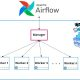2021-12-26
###### Theory of Generalization: growth function, dichotomies, and break points
2022-01-11

The bias-variance trade-off is an important concept in statistics and machine learning. This is used to get better performance out of machine learning models. To understand this concept we must understand the meaning of the terms Bias and Variance.

The total error of a model can be decomposed into:

1. Reducible Error
2. Irreducible Error

Bias and variance are reducible errors. The irreducible error refers to the error that can not be reduced, often known as noise. So, even the best models will always have an error that can not be removed. Hence there is a trade-off between bias and variance to decrease the reducible error which in turn would minimize the total error.

# What is bias?

The inability of a model to accurately capture the true relationship is called bias. Models with high bias are simple and fail to capture the complexity of the data. Hence, such models lead to higher training and testing errors. Low bias corresponds to a good fit to the training dataset. Generally, more flexible models result in lower bias.

# What is variance?

Variance refers to the amount by which the estimate of the true relationship would change on using a different training dataset. Using different training sets will result in different estimations but ideally, the estimate should not vary much over different training sets. High variance implies that the model does not perform well on previously unseen data (testing data) even if it fits the training data well. Generally, more flexible models have higher variance. Low variance implies that the model performs well on the testing set.

# Overfitting and Underfitting

Overfitting occurs when a model captures the noise along with the data pattern. A model that fits the training data well but fails to do so on the testing set is an overfit to the data. Overfitted models have low bias and high variance.

Underfitting occurs when a model fails to even capture the pattern of the data. Such models have high bias and low variance.

This is a way to make sure that the model is neither overfitted nor under fitted. Ideally, a model should have low bias so that it can accurately model the true relationship and low variance so that it can produce consistent results and perform well on testing data. This is called a trade-off as it is challenging to find a model for which both the bias and variance are low.

# Mathematical view

The total error can be written as a mathematical equation:

This equation suggests that we need to find a model that simultaneously achieves low bias and low variance. Variance is a non-negative term and bias squared is also non-negative which implies the total error can never go below the irreducible error.

This graph suggests that as we increase model complexity (flexibility), the bias initially decreases faster than variance increases. Consequently, the total error decreases. However, at one point, increasing the model complexity has little effect on the bias but the variance increases significantly. Consequently, the total error also increases. Therefore, this point is the optimal point for minimum total error.

# Derivation of the Equation

Let’s start by defining the various notations used. We have independent variables x that affect the value of a dependent variable y. Function denotes the true relationship between x and y. In real-life problems, it is very hard to know this relationship. is given by this formula along with some noise which is represented by the random variable ϵ with zero mean and variance (sigma_ϵ)²:

Mathematically, ϵ has the following properties:

Now, when we try to model the underlying real-life problem, we try to find a function f̂ that can accurately predict the true relationship f. The goal is to bring the prediction as close as possible to the actual value (y ≈ f̂(x)) to minimize the error.

Now, coming to the bias-variance trade-off equation:

Here, E[(f̂(x))²] is the Mean Squared Error, commonly known as MSE. This is defined as the average squared difference of a prediction f̂(x) from its true value y.

Bias is defined as the difference between the average value of prediction from the true relationship function f(x).

Variance is defined as the expectation of the squared deviation of f̂(x) from its expected value E[f̂(x)].

Starting from the LHS of the equation, E[(f̂(x))²]:

Replacing y by f(x)+ϵ in the first line, we proceed by expanding further using the linear property of expectation and independence of the random variables ϵ and . Then using the properties of ϵ and the fact that hat when two random variables are independent, the expectation of their product is equal to the product of their expectations.

Now, by further expanding the term on the RHS, E[(f(x) f̂(x))²]:

E[f̂(x)] − f(x) is a constant since we subtract f(x), a constant from E[f̂(x)] which is also a constant. So, E[(E[f̂(x)] − f(x))²] = (E[f̂(x)] − f(x))². Further expanding using the linearity property of expectation we get the value of E[(f(x) f̂(x))²]. Plugging this value back into the equation for E[(f̂(x))²], we arrive on our final equation:

References: